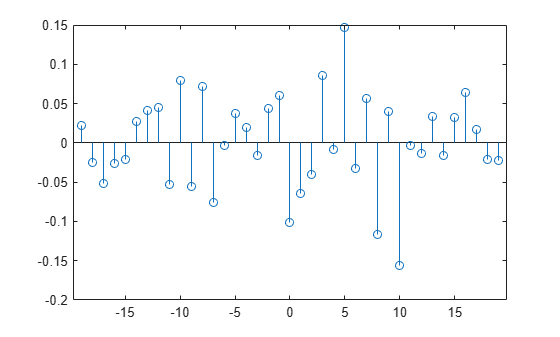Main Content

# Create Composite System object

This example shows how to create a System object™ composed of other System objects. This System object uses two moving average System objects to find the cross-correlation of two independent samples. The Create Moving Average System object example explains in detail how to create a System object. This example focuses on how to use a System object within another System object.

### System objects as Private Properties

The ability to create more than one instance of a System object and having each instance manage its own state is one of the biggest advantages of using System objects over functions. The private properties `MovingAverageFilter1` and `MovingAverageFilter2` are used to store the two moving average filter objects.

```properties (Access=private) % This example class contains two moving average filters (more can be added % in the same way) MovingAverageFilter1 MovingAverageFilter2 end ```

### Set Up the Moving Average Filter

In the `setupImpl` method, create the two moving average System objects and initialize their public properties.

```function setupImpl(obj,~) % Set up moving average objects with default values obj.MovingAverageFilter1 = movingAverageFilter('WindowLength',obj.WindowLength1); obj.MovingAverageFilter2 = movingAverageFilter('WindowLength',obj.WindowLength2); end ```

### Work with Dependent Properties

The `WindowLength` public property from the movingAverage`Filter` System object is implemented as a dependent property in this example.

```properties(Nontunable,Dependent) % WindowLength Moving window length WindowLength1; WindowLength2; end ```

Whenever you assign a value to one of the dependent properties, the value is set in the corresponding moving average filter. When you read one of the dependent properties, the value is read from the corresponding moving average filter.

```function set.WindowLength1(obj,WindowLength1) % Set the window length of one moving average filter obj.MovingAverageFilter1.WindowLength = WindowLength1; end function WindowLength = get.WindowLength1(obj) % Read window length from one of the moving average filters WindowLength = obj.MovingAverageFilter1.WindowLength; end function set.WindowLength2(obj,WindowLength2) % Set the window length of one moving average filter obj.MovingAverageFilter2.WindowLength = WindowLength2; end function WindowLength = get.WindowLength2(obj) % Read window length from one of the moving average filters WindowLength = obj.MovingAverageFilter2.WindowLength; end ```

### Use the Cross-Correlation Object in MATLAB

Create random variables to calculate the cross-correlation of their moving averages, then view the results in a stem plot.

```x = rand(20,1); y = rand(20,1); crossCorr = crossCorrelationMovingAverages('WindowLength1',1,'WindowLength2',5); for iter = 1:100 x = rand(20,1); y = rand(20,1); [corr,lags] = crossCorr(x,y); stem(lags,corr) end```Download ebook